# 谱线是在哪里形成的？

## 谱线的贡献函数：一堆老论文

Posted by mingjie on August 4, 2019

## 我们要解决什么问题？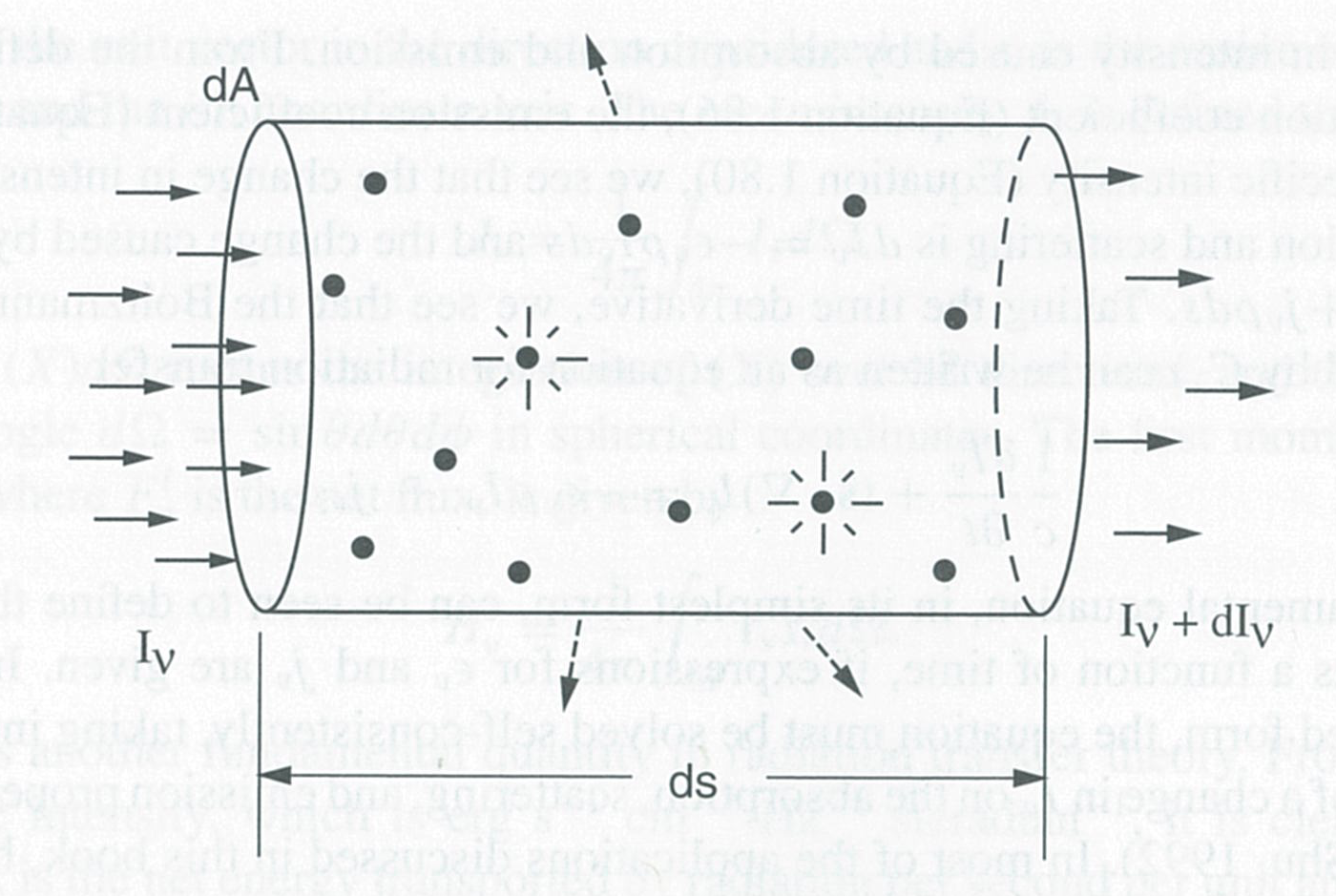• 星光怎么是外部光源：这里的模型是恒星的连续谱是从一个内部的球面发出来的，然后打到球面外面的大气层里面；这个模型肯定不怎么准确（并且不同的波长上连续谱的位置也不一样），但是理解来说够用了。
• 管子一定处于恒星大气中吗：不一定。最极端的例子是telluric线，它的连续谱是恒星，但是吸收（也就是形成）的区域是在地球大气上，一个天一个地。

## 光强减少的贡献函数

$R_I$$z$求导，有：

## 流量减少的贡献函数？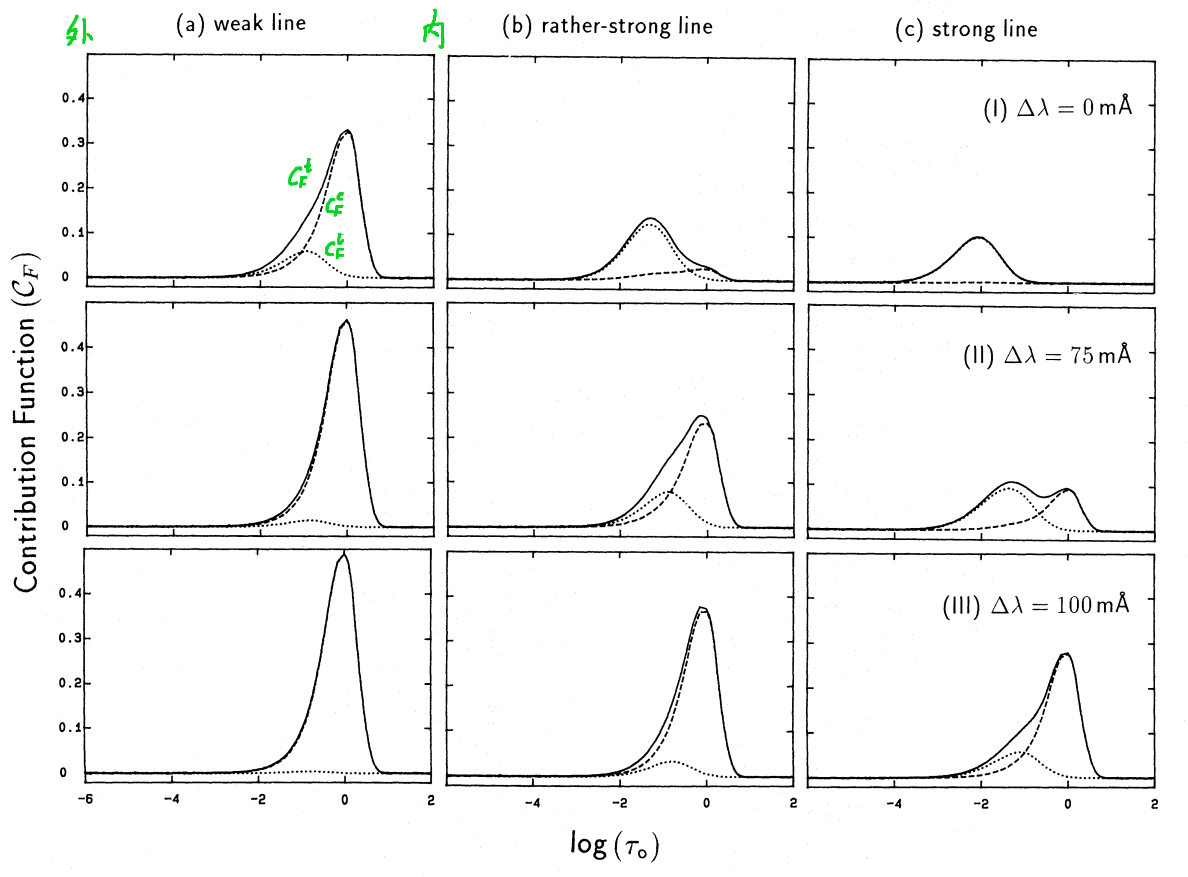Achmad 1991 图2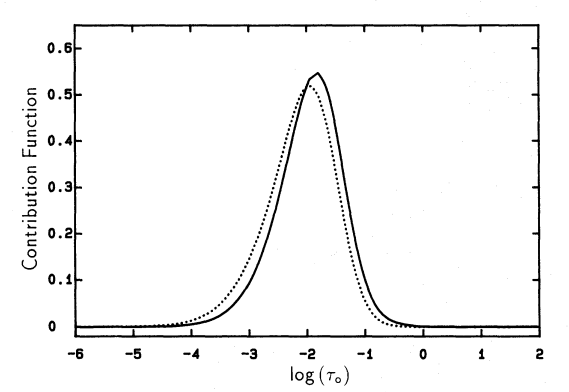Achmad 1991 图3

## 究竟哪些能用哪些不能用？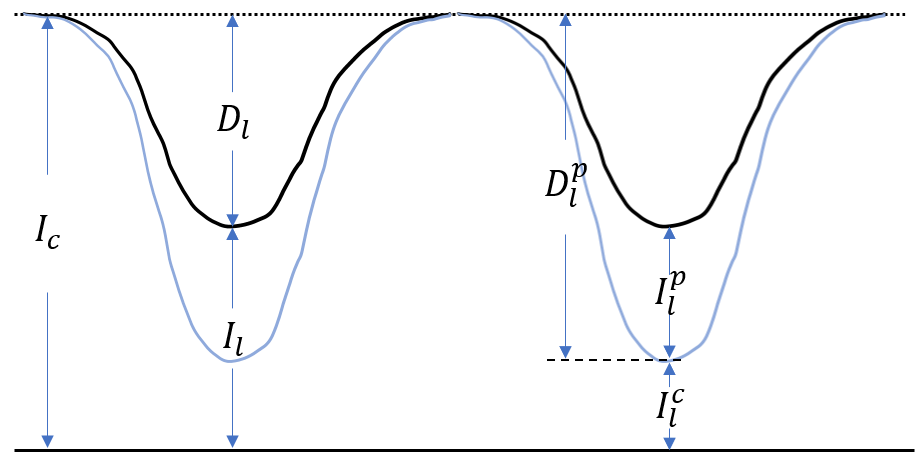Gurtovenko+1991 图1

1. $I_c$是连续谱的发射，代表着谱线不存在时的出射光强
2. $D_l^p$是连续谱发射通过谱线区域时被谱线吸收的量（纯吸收，谱线的发射不计算在内）
3. $I_l^c$是连续谱发射通过谱线区域时没有被吸收的量，也就是最终谱线轮廓处连续谱光强占的比例
4. $I_l^p$是谱线发射光强占的比例（谱线发射在这里被count了）
5. $D_l = D_l^p - I_l^p$是最终观测到的谱线总吸收
6. $I_l = I_l^c + I_l^p$是最终观测到的谱线总发射光强

### $D_l^p$

$I_c$的定义代入并定义$\eta = \kappa_l / \kappa_c$以及$\kappa_l(\tau_c) \Delta h(\tau_c) = \eta(\tau_c) \Delta\tau_c$，有：

### $I_l$

$I_l = I_l^c + I_l^p$，有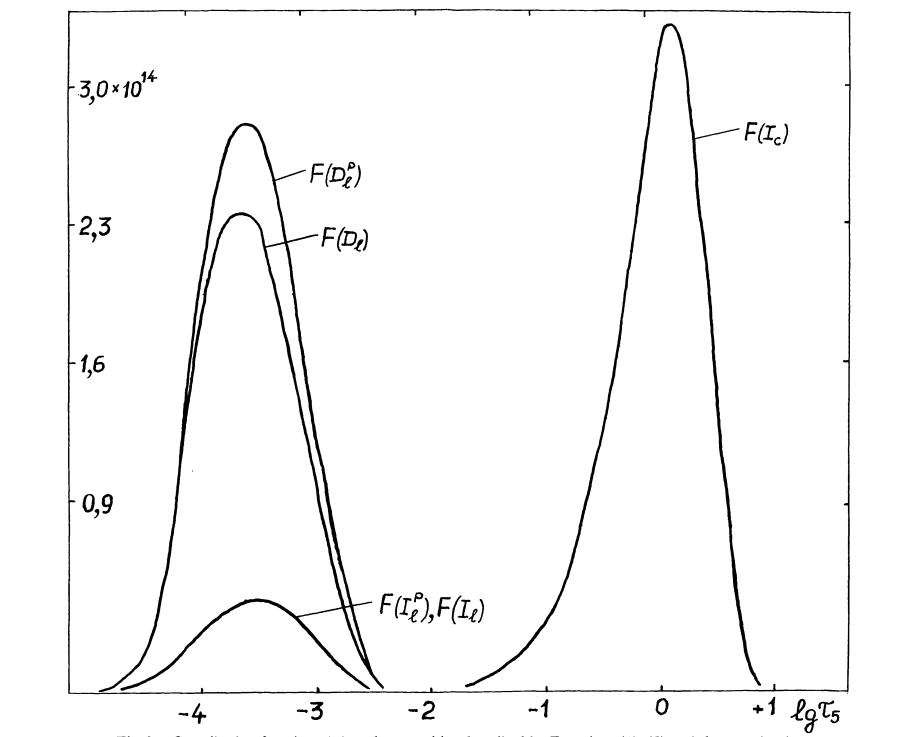Gurtovenko+1991 图2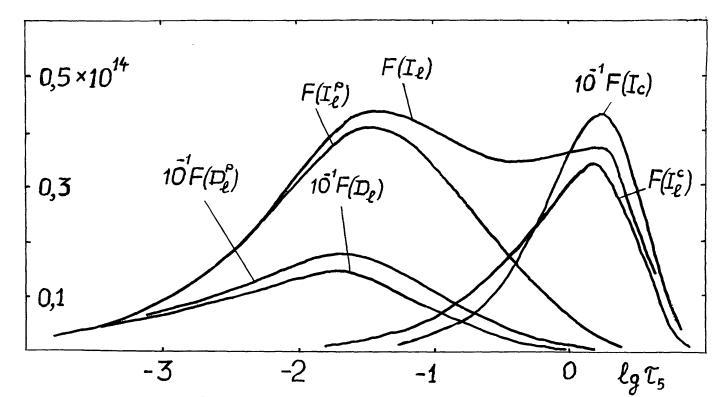Gurtovenko+1991 图4

## 贡献函数和恒星参数的关系

### 速度场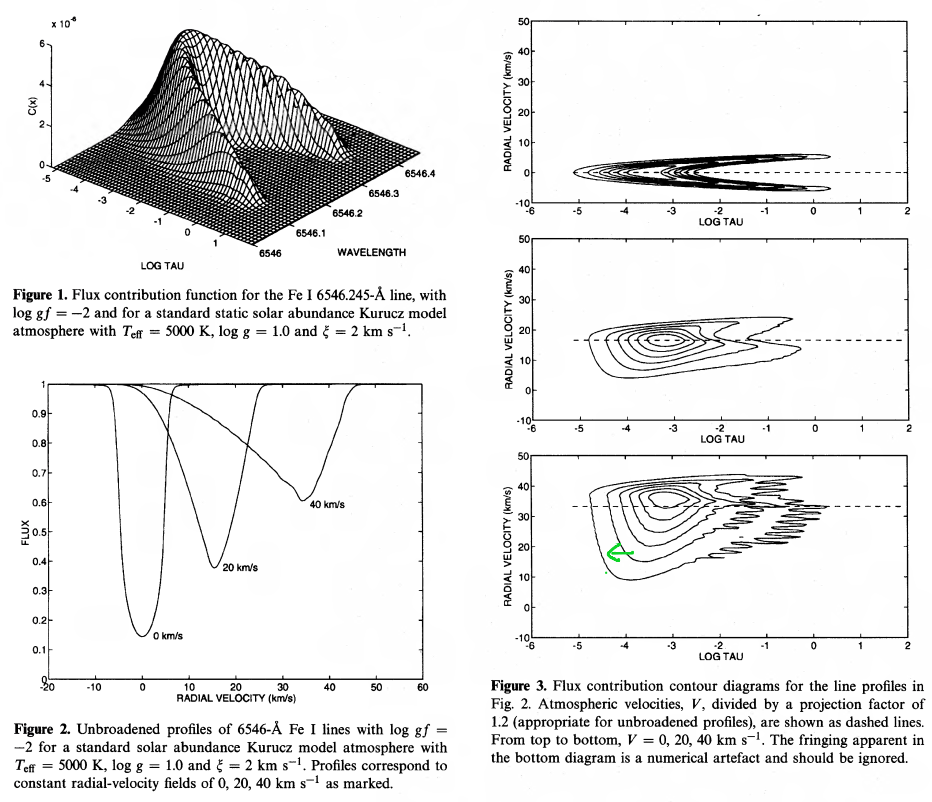Albow+1996

### 激发能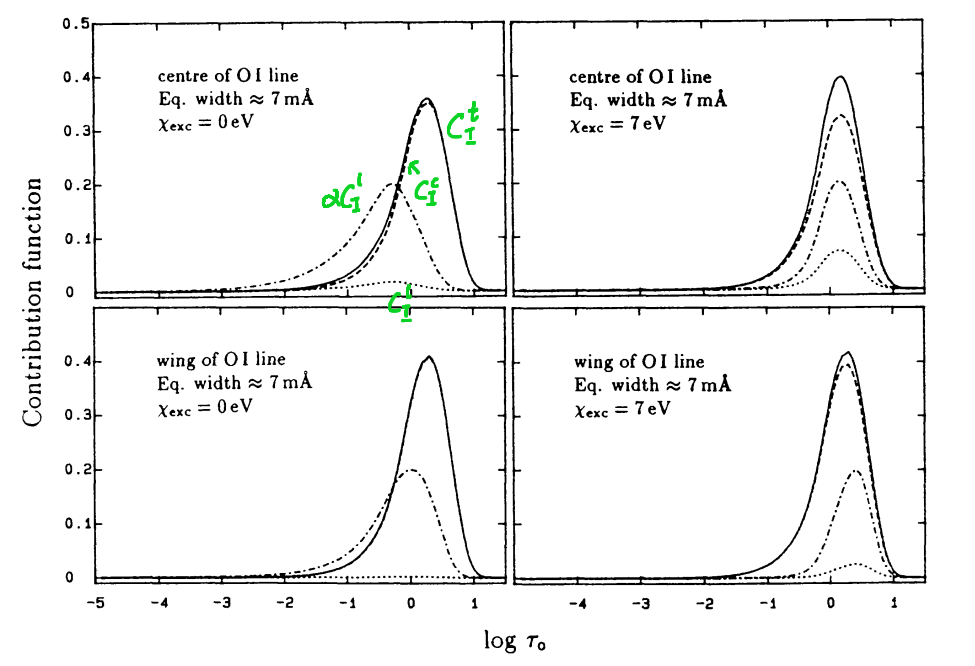Achmad 1992 图2

### 3D-NLTE下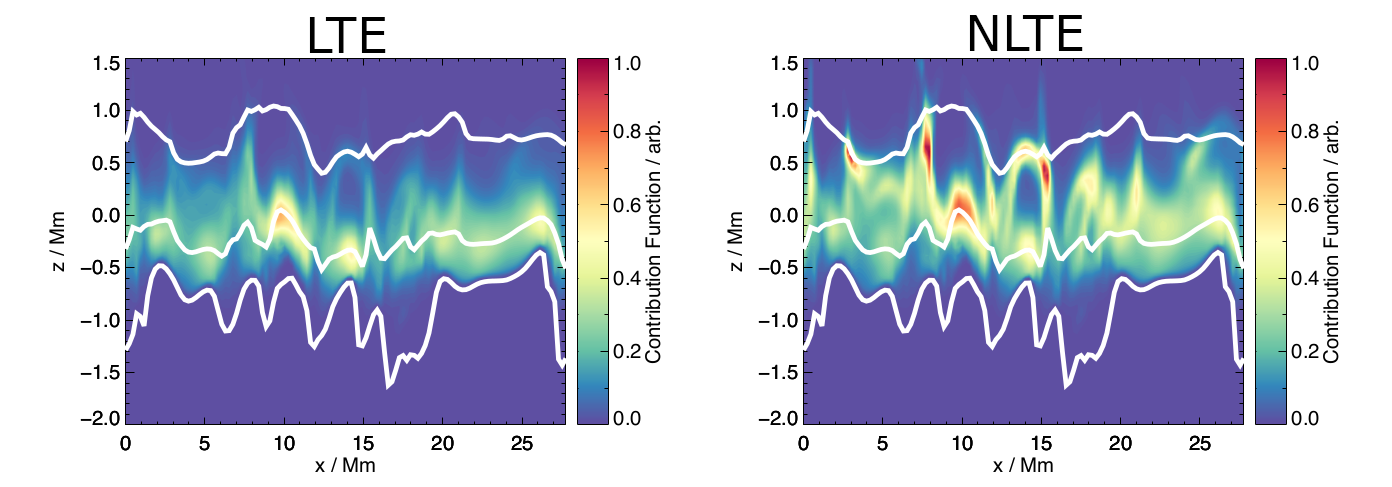Amarsi 2015 图3

### 恒星大气切片